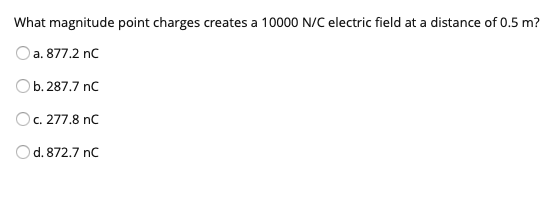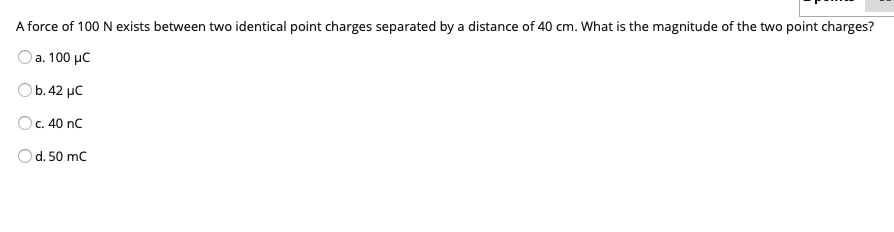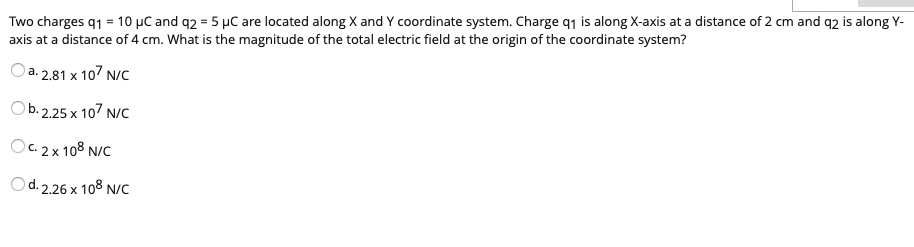# Question Solved1 AnswerWhat magnitude point charges creates a 10000 N/C electric field at a distance of 0.5 m? a. 877.2 nc Ob. 287.7 nc Oc. 277.8 nc O d. 872.7 nc A force of 100 N exists between two identical point charges separated by a distance of 40 cm. What is the magnitude of the two point charges? O a. 100 LC b.42 pc O c. 40 nc Od. 50 mc Two charges 91 = 10 C and 92 = 5 pc are located along X and Y coordinate system. Charge q1 is along X-axis at a distance of 2 cm and q2 is along Y- axis at a distance of 4 cm. What is the magnitude of the total electric field at the origin of the coordinate system? a. 2.81 x 107 NC Ob.2.25 x 107 N/C Oc 2x 108 NC d. 2.26 x 108 N/CTranscribed Image Text: What magnitude point charges creates a 10000 N/C electric field at a distance of 0.5 m? a. 877.2 nc Ob. 287.7 nc Oc. 277.8 nc O d. 872.7 nc A force of 100 N exists between two identical point charges separated by a distance of 40 cm. What is the magnitude of the two point charges? O a. 100 LC b.42 pc O c. 40 nc Od. 50 mc Two charges 91 = 10 C and 92 = 5 pc are located along X and Y coordinate system. Charge q1 is along X-axis at a distance of 2 cm and q2 is along Y- axis at a distance of 4 cm. What is the magnitude of the total electric field at the origin of the coordinate system? a. 2.81 x 107 NC Ob.2.25 x 107 N/C Oc 2x 108 NC d. 2.26 x 108 N/C
More
Transcribed Image Text: What magnitude point charges creates a 10000 N/C electric field at a distance of 0.5 m? a. 877.2 nc Ob. 287.7 nc Oc. 277.8 nc O d. 872.7 nc A force of 100 N exists between two identical point charges separated by a distance of 40 cm. What is the magnitude of the two point charges? O a. 100 LC b.42 pc O c. 40 nc Od. 50 mc Two charges 91 = 10 C and 92 = 5 pc are located along X and Y coordinate system. Charge q1 is along X-axis at a distance of 2 cm and q2 is along Y- axis at a distance of 4 cm. What is the magnitude of the total electric field at the origin of the coordinate system? a. 2.81 x 107 NC Ob.2.25 x 107 N/C Oc 2x 108 NC d. 2.26 x 108 N/C